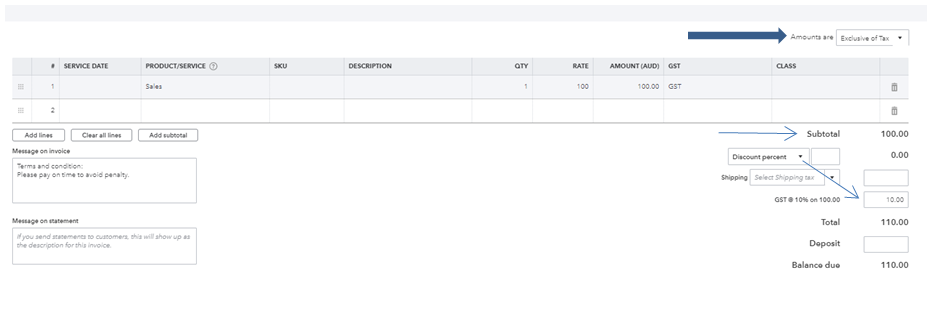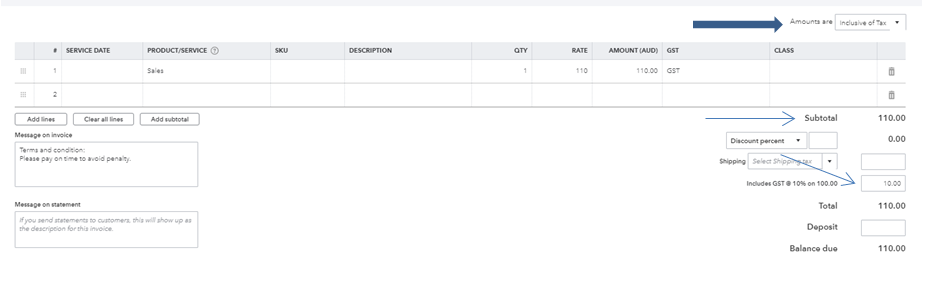cancel
Showing results for
Did you mean:Level 2

# What is the difference between tax exclusive and tax inclusive?

What is the difference between tax exclusive and tax inclusive?

SolvedLevel 2

## What is the difference between tax exclusive and tax inclusive?

Tax-exclusive

This refers to the amount of tax paid as a proportion of the pretax value of whatever is taxed.To calculate how much GST to add, multiply by 0.1.

Tax-inclusive

This refers to the amount of tax paid as a proportion of the after-tax value.To calculate how much GST is included in a price, Divide by 11.

I hope this helps!

1 Comment 1Level 2

## What is the difference between tax exclusive and tax inclusive?

Tax-exclusive

This refers to the amount of tax paid as a proportion of the pretax value of whatever is taxed.To calculate how much GST to add, multiply by 0.1.

Tax-inclusive

This refers to the amount of tax paid as a proportion of the after-tax value.To calculate how much GST is included in a price, Divide by 11.

I hope this helps!

Sitemap